# VBA Right FunctionArticle byJeevan A Y## What is Right Function in VBA Excel?

VBA Right Function is a text function that helps us extract a specified number of characters from the right side of a given string or text. For example, the VBA Right function will extract characters from right to left.

One of the most common examples of using the VBA RIGHT function is to extract the last name from the full name available. For example, look at the following data in Excel. We have the full name “Michael Clarke” in cell A2. Therefore, we must use the RIGHT function to extract the last name, as shown in the following code.

Once we execute the code, it will extract the last name and store it in cell B2.

###### Key Takeaways
• The VBA RIGHT function returns a substring from the right side of the string based on the number of characters given in the length argument of the function.
• The VBA RIGHT function uses the Instr and LEN functions to retrieve a substring from the full string dynamically.
• With the help of the FOR LOOP, the VBA RIGHT function can loop through all the cells and extract substrings from all the cells dynamically.

### How to Use Right Function in VBA Excel?

Before applying the function, let us show you, its syntax. The following image shows the syntax of the VBA Right Function.

The function has two arguments, and both are mandatory.

• String: The string value from which we will look to extract the substring.
• Length: The number of characters to be extracted from the right side of the given string.

For example, if the string is “Excel VBA” and we want to extract the substring “VBA,” then we can give the String argument as “Excel VBA” and length as three because VBA has three characters.

Now let us look at a basic example of applying the VBA RIGHT function.

We have a text, “Sydney Sixers,” in one of the Excel cells. Assume we must extract the last name “Sixers.” The following steps are listed below.

1. Open the Visual Basic Editor (VBE) window by pressing the ALT + F11 shortcut key from the Excel worksheet.

2. Once the Visual Basic Editor (VBE) window is opened, go to the Insert tab and click on “Module” to insert a new module.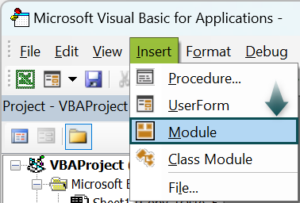3. Double-click on the “Module.” You will see coding space on the right side. Start the sub-procedure by naming the macro.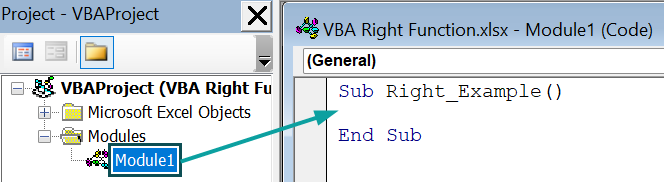4. Inside the sub-procedure, we will write the code. Since we must extract the last name to cell B2, we will reference the cell using the RANGE object with its Value property. We have entered an equal sign because we set the value for cell B2.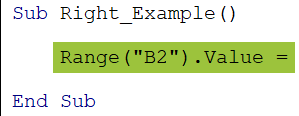5. Enter the VBA RIGHT function after the equal to sign.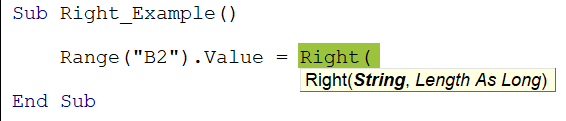6. For the string argument of the RIGHT function, give the value of the cell A2. Let us use the RANGE Object with its Value property, as shown below.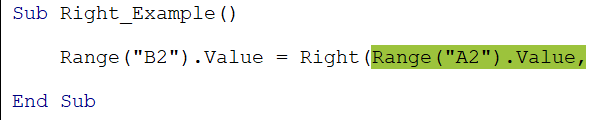7. For the length argument, we must give the number of characters to be extracted from the right side of the given string.

In this scenario, we must extract the substring “Sixers,” which has six characters. Hence, enter the length as 6.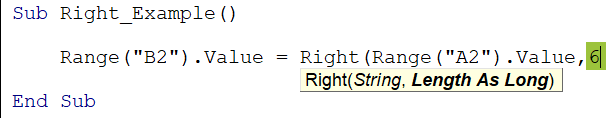8. Now, close the bracket and execute the code by pressing the shortcut key “F5.” We get the value “Sixers” in cell B2.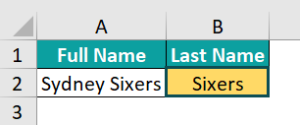### Examples of Excel VBA Right Function

We will show you some practical examples of applying the VBA Right Function in the examples below.

#### Example #1 – Extract Specified Number of Characters

To use the VBA RIGHT function correctly, we must first understand its complete functionality. For example, assuming we have a string “Wallstreet Mojo,” let us try to extract a single character at a time and see how it works.

• Step 1: First enter the value in an Excel cell, A1 in this case.
• Step 2: In the sub-procedure, define a variable to hold the cell value.
• Step 3: Assign the value in the cell A2 to this variable.
• Step 4: Define another variable to assign the value extracted from RIGHT.
• Step 5: Assign the VBA RIGHT function to this variable.
• Step 6: For the first argument of the VBA RIGHT function, give the variable name “FullString,” which holds the entire string value i.e., “Wallstreet Mojo.”
• Step 7: For the length argument, let’s enter one now.
• Step 8: Store the RIGHT function variable value in cell B2.

When we run the code, we see the following value extracted in cell B2.

Since we have given the length as 1, the VBA RIGHT function has extracted only one character from the right side of the full string, i.e., “o”.

Similarly, now change the length from 1 to 2.

Now the RIGHT function has extracted two characters.

Similarly, play around with several characters, and you should be able to extract a given number of characters from the right side of the full string.

#### Example #2

Here, we have an entire string, “P4563 Commercial.” If we want to extract the second portion of the string, i.e., “Commercial,” we can provide the length as ten because the substring “Commercial” has ten characters.

• Part 1: We have defined a variable and assigned a value.
• Part 2: We have defined another variable, applied the VBA RIGHT function, and extracted ten characters from the right side of the string.
• Part 3: We are showing the extracted value in a message box.

When we execute the above code, we will get the following result in a VBA message box.

However, the drawback of this hard coding of the number of characters to be extracted is that it is not dynamic. For example, assume we have a string “P3890 Party” and specify the number of characters as 10.

We get the following result.

This time we have not only got the substring but the first portion of the string as well. Again, this is because of the number of characters specified, i.e., 10.

We can use the dynamic approach explained in the article’s next section to avoid hard coding.

### Dynamic RIGHT Function in Excel VBA

#### RIGHT Function with INSTR

For the VBA RIGHT function to work dynamically, i.e., to get the number of characters from different strings automatically without worrying about the length of the substring to be extracted, the VBA function “Instr” plays a vital role.

The Instr function in VBA helps us get the position of a given character in a string. So, for example, if we have the string “Lenovo Laptop” and have to find the position of the character “o” from the beginning, we can use the Instr function.

The crucialpart of the code is InStr(1, FullString, “o”).

Instr function starting from position one will look for the character “o” in the string “Lenovo Laptop.”

When we run this VBA code, we will get the character “o” position in a message box.

The character “o” is in the 4th position in the string “Lenovo Laptop” starting from first position.

However, let’s change the code’s starting position from 1 to 5.

Now, we will get the following result in a message box.

Here, the position of the character “o” changed from 4 to 6 because the starting position in the Instr function has been changed to 5. Hence, it ignored the 4th character “o” and started searching for the character “o” only from the 5th position onwards.

#### RIGHT Function with LEN

Another function that can be used to assist the VBA RIGHT function is the LEN function. The LEN function helps us to find the total length of the string. For example, the string “Lenovo Laptop” has 13 characters, including the space. The LEN function helps us find the total characters of a given string. Combining these two functions allows us to extract the total number of characters from the right side of the string.

For instance, look at the following example.

• String: Lenovo Laptop
• Total Characters: 13
• Space Character Position: 7
• Total Characters to be extracted from right side: 13 – 7 = 6.

Using this logic, we can dynamically get the number of characters extracted from the right side of the string. For example, the following code will dynamically extract the right side of the string.

This will dynamically extract the second part of the string i.e., “Laptop”.

Change the variable “Full_String” value; we should get the substring without changing anything. For example, let’s assign the value “JP Morgan.”

Now we will dynamically get the value “Morgan” in the message box.

#### Loops with Right Function in Excel VBA

Thus far, we have applied a single value to the VBA RIGHT function. However, when multiple values are in the Excel worksheet, we cannot write the code for each cell value. For example, look at the following data in Excel.

We have the Code and Product in column A. Therefore, in column B we have to extract only the Product name from column A. To do this, we need not write multiple VBA RIGHT functions. Instead, we will use a single RIGHT function inside the FOR LOOP to go through all the cells and extract the product names dynamically from them.

This code will dynamically find the last used cell (in column A) and find the number of characters to be extracted from the right side of the string.

Once we run the code, it will extract substrings from all the available values.

### Important Things to Note

• If the length argument is omitted, the VBA Right function will return a compile error.
• The VBA RIGHT function not working occurs if the length argument value is non-numeric and will result in a type mismatch error.
• INSTR becomes a case-sensitive search if the vbBinaryCompare is used.
• The VBA RIGHT function considers space as a character.

1. Why RIGHT function in VBA Excel is not working?

The VBA RIGHT function will return a compile error if the length argument of the RIGHT function is not given any values.
For example, look at the following code.We have not given any value for the length argument in the above code after the string argument. Therefore, we will get the following compile error when executing the code.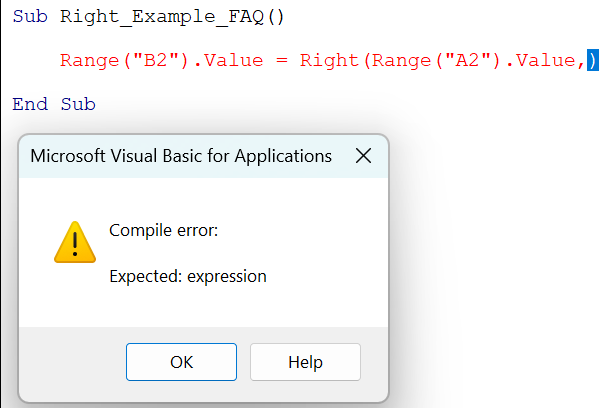2. What is the purpose of RIGHT function in Excel VBA?

The VBA RIGHT function extracts a substring of a given string, which will be extracted from the right side of the full string.

3. What is the difference between LEFT and RIGHT function in Excel VBA?

• LEFT: This will extract the substring from the left side of the full string.
RIGHT: This will extract the substring from the right side of the full string.